# How To Understand Derivatives: The Product, Power & Chain Rules

The jumble of rules for taking derivatives never truly clicked for me. The addition rule, product rule, quotient rule -- how do they fit together? What are we even trying to do?

Here's my take on derivatives:

• We have a system to analyze, our function f
• The derivative f' (aka df/dx) is the moment-by-moment behavior
• It turns out f is part of a bigger system (h = f + g)
• Using the behavior of the parts, can we figure out the behavior of the whole?

Yes. Every part has a "point of view" about how much change it added. Combine every point of view to get the overall behavior. Each derivative rule is an example of merging various points of view.

And why don't we analyze the entire system at once? For the same reason you don't eat a hamburger in one bite: small parts are easier to wrap your head around.

Instead of memorizing separate rules, let's see how they fit together: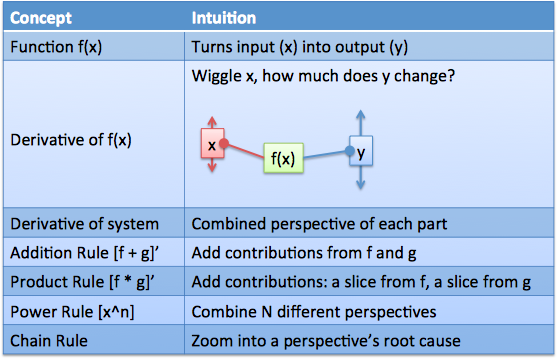The goal is to really grok the notion of "combining perspectives". This installment covers addition, multiplication, powers and the chain rule. Onward!

## Functions: Anything, Anything But Graphs

The default calculus explanation writes "f(x) = x^2" and shoves a graph in your face. Does this really help our intuition?

Not for me. Graphs squash input and output into a single curve, and hide the machinery that turns one into the other. But the derivative rules are about the machinery, so let's see it!

I visualize a function as the process "input(x) => f => output(y)".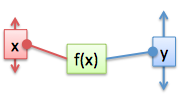It's not just me. Check out this incredible, mechanical targetting computer (beginning of youtube series).

The machine computes functions like addition and multiplication with gears -- you can see the mechanics unfolding!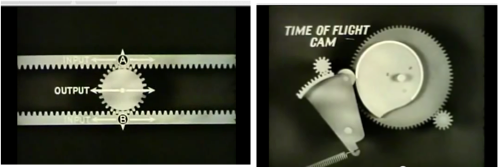Think of function f as a machine with an input lever "x" and an output lever "y". As we adjust x, f sets the height for y. Another analogy: x is the input signal, f receives it, does some magic, and spits out signal y. Use whatever analogy helps it click.

## Wiggle Wiggle Wiggle

The derivative is the "moment-by-moment" behavior of the function. What does that mean? (And don't mindlessly mumble "The derivative is the slope". See any graphs around these parts, fella?)

The derivative is how much we wiggle. The lever is at x, we "wiggle" it, and see how y changes. "Oh, we moved the input lever 1mm, and the output moved 5mm. Interesting."

The result can be written "output wiggle per input wiggle" or "dy/dx" (5mm / 1mm = 5, in our case). This is usually a formula, not a static value, because it can depend on your current input setting.

For example, when f(x) = x^2, the derivative is 2x. Yep, you've memorized that. What does it mean?

If our input lever is at x = 10 and we wiggle it slightly (moving it by dx=0.1 to 10.1), the output should change by dy. How much, exactly?

• We know f'(x) = dy/dx = 2 * x
• At x = 10 the "output wiggle per input wiggle" is = 2 * 10 = 20. The output moves 20 units for every unit of input movement.
• If dx = 0.1, then dy = 20 * dx = 20 * .1 = 2

And indeed, the difference between 10^2 and (10.1)^2 is about 2. The derivative estimated how far the output lever would move (a perfect, infinitely small wiggle would move 2 units; we moved 2.01).

The key to understanding the derivative rules:

• Set up your system
• Wiggle each part of the system separately, see how far the output moves
• Combine the results

The total wiggle is the sum of wiggles from each part.

## Addition and Subtraction

Time for our first system: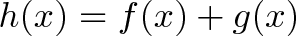$\displaystyle{h(x) = f(x) + g(x) }$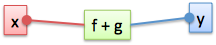What happens when the input (x) changes?

In my head, I think "Function h takes a single input. It feeds the same input to f and g and adds the output levers. f and g wiggle independently, and don't even know about each other!"

Function f knows it will contribute some wiggle (df), g knows it will contribute some wiggle (dg), and we, the prowling overseers that we are, know their individual moment-by-moment behaviors are added: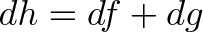$\displaystyle{dh = df + dg}$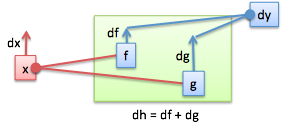Again, let's describe each "point of view":

• The overall system has behavior dh
• From f's perspective, it contributes df to the whole [it doesn't know about g]
• From g's perspective, it contributes dg to the whole [it doesn't know about f]

Every change to a system is due to some part changing (f and g). If we add the contributions from each possible variable, we've described the entire system.

## df vs df/dx

Sometimes we use df, other times df/dx -- what gives? (This confused me for a while)

• df is a general notion of "however much f changed"
• df/dx is a specific notion of "however much f changed, in terms of how much x changed"

The generic "df" helps us see the overall behavior.

An analogy: Imagine you're driving cross-country and want to measure the fuel efficiency of your car. You'd measure the distance traveled, check your tank to see how much gas you used, and finally do the division to compute "miles per gallon". You measured distance and gasoline separately -- you didn't jump into the gas tank to get the rate on the go!

In calculus, sometimes we want to think about the actual change, not the ratio. Working at the "df" level gives us room to think about how the function wiggles overall. We can eventually scale it down in terms of a specific input.

And we'll do that now. The addition rule above can be written, on a "per dx" basis, as: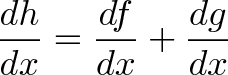$\displaystyle{\frac{dh}{dx} = \frac{df}{dx} + \frac{dg}{dx}}$

## Multiplication (Product Rule)

Next puzzle: suppose our system multiplies parts "f" and g". How does it behave?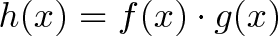$\displaystyle{h(x) = f(x) \cdot g(x)}$

Hrm, tricky -- the parts are interacting more closely. But the strategy is the same: see how each part contributes from its own point of view, and combine them:

• total change in h = f's contribution (from f's point of view) + g's contribution (from g's point of view)

Check out this diagram: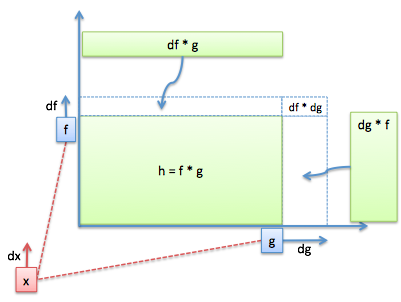What's going on?

• We have our system: f and g are multiplied, giving h (the area of the rectangle)
• Input "x" changes by dx off in the distance. f changes by some amount df (think absolute change, not the rate!). Similarly, g changes by its own amount dg. Because f and g changed, the area of the rectangle changes too.
• What's the area change from f's point of view? Well, f knows he changed by df, but has no idea what happened to g. From f's perspective, he's the only one who moved and will add a slice of area = df * g
• Similarly, g doesn't know how f changed, but knows he'll add as slice of area "dg * f"

The overall change in the system (dh) is the two slices of area: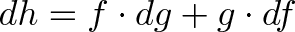$\displaystyle{dh = f \cdot dg + g \cdot df}$

Now, like our miles per gallon example, we "divide by dx" to write this in terms of how much x changed: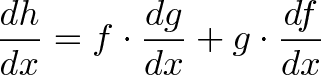$\displaystyle{\frac{dh}{dx} = f \cdot \frac{dg}{dx} + g \cdot \frac{df}{dx}}$

(Aside: Divide by dx? Engineers will nod, mathematicians will frown. Technically, df/dx is not a fraction: it's the entire operation of taking the derivative (with the limit and all that). But infinitesimal-wise, intuition-wise, we are "scaling by dx". I'm a smiler.)

The key to the product rule: add two "slivers of area", one from each point of view.

Gotcha: But isn't there some effect from both f and g changing simultaneously (df * dg)?

Yep. However, this area is an infinitesimal * infinitesimal (a "2nd-order infinitesimal") and invisible at the current level. It's a tricky concept, but (df * dg) / dx vanishes compared to normal derivatives like df/dx. We vary f and g indepdendently and combine the results, and ignore results from them moving together.

## The Chain Rule: It's Not So Bad

Let's say g depends on f, which depends on x: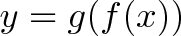$\displaystyle{y = g(f(x))}$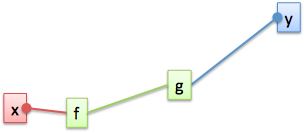The chain rule lets us "zoom into" a function and see how an initial change (x) can effect the final result down the line (g).

Interpretation 1: Convert the rates

A common interpretation is to multiply the rates: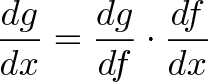$\displaystyle{\frac{dg}{dx} = \frac{dg}{df} \cdot \frac{df}{dx}}$

x wiggles f. This creates a rate of change of df/dx, which wiggles g by dg/df. The entire wiggle is then: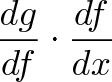$\displaystyle{\frac{dg}{df} \cdot \frac{df}{dx}}$

This is similar to the "factor-label" method in chemistry class: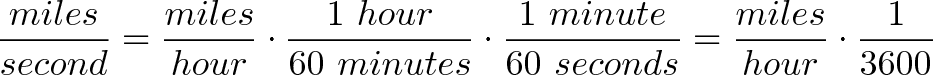$\displaystyle{\frac{miles}{second} = \frac{miles}{hour} \cdot \frac{1 \ hour}{60 \ minutes} \cdot \frac{1 \ minute}{60 \ seconds} = \frac{miles}{hour} \cdot \frac{1}{3600}}$

If your "miles per second" rate changes, multiply by the conversion factor to get the new "miles per hour". The second doesn't know about the hour directly -- it goes through the second => minute conversion.

Similarly, g doesn't know about x directly, only f. Function g knows it should scale its input by dg/df to get the output. The initial rate (df/dx) gets modified as it moves up the chain.

Interpretation 2: Convert the wiggle

I prefer to see the chain rule on the "per-wiggle" basis:

• x wiggles by dx, so
• f wiggles by df, so
• g wiggles by dg

Cool. But how are they actually related? Oh yeah, the derivative! (It's the output wiggle per input wiggle):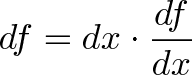$\displaystyle{df = dx \cdot \frac{df}{dx}}$

Remember, the derivative of f (df/dx) is how much to scale the initial wiggle. And the same happens to g: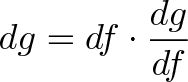$\displaystyle{dg = df \cdot \frac{dg}{df}}$

It will scale whatever wiggle comes along its input lever (f) by dg/df. If we write the df wiggle in terms of dx: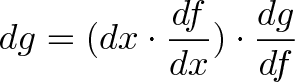$\displaystyle{dg = (dx \cdot \frac{df}{dx}) \cdot \frac{dg}{df}}$

We have another version of the chain rule: dx starts the chain, which results in some final result dg. If we want the final wiggle in terms of dx, divide both sides by dx: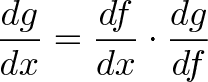$\displaystyle{\frac{dg}{dx} = \frac{df}{dx} \cdot \frac{dg}{df}}$

The chain rule isn't just factor-label unit cancellation -- it's the propagation of a wiggle, which gets adjusted at each step.

The chain rule works for several variables (a depends on b depends on c), just propagate the wiggle as you go.

Try to imagine "zooming into" different variable's point of view. Starting from dx and looking up, you see the entire chain of transformations needed before the impulse reaches g.

## Chain Rule: Example Time

Let's say we put a "squaring machine" in front of a "cubing machine":

input(x) => f:x^2 => g:f^3 => output(y)

f:x^2 means f squares its input. g:f^3 means g cubes its input, the value of f. For example:

input(2) => f(2) => g(4) => output:64

Start with 2, f squares it (2^2 = 4), and g cubes this (4^3 = 64). It's a 6th power machine: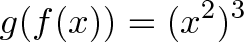$\displaystyle{g(f(x)) = (x^2)^3}$

And what's the derivative?$\displaystyle{ \frac{dg}{dx} = \frac{dg}{df} \cdot \frac{df}{dx}}$

• f changes its input wiggle by df/dx = 2x
• g changes its input wiggle by dg/df = 3f^2

The final change is: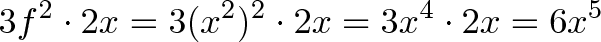$\displaystyle{3f^2 \cdot 2x = 3(x^2)^2 \cdot 2x = 3x^4 \cdot 2x = 6x^5}$

## Chain Rule: Gotchas

Functions treat their inputs like a blob

In the example, g's derivative ("x^3 = 3x^2") doesn't refer to the original "x", just whatever the input was (foo^3 = 3*foo^2). The input was f, and it treats f as a single value. Later on, we scurry in and rewrite f in terms of x. But g has no involvement with that -- it doesn't care that f can be rewritten in terms of smaller pieces.

In many examples, the variable "x" is the "end of the line".

Questions ask for df/dx, i.e. "Give me changes from x's point of view". Now, x could depend on something deeper variable, but that's not being asked for. It's like saying "I want miles per hour. I don't care about miles per minute or miles per second. Just give me miles per hour". df/dx means "stop looking at inputs once you get to x".

How come we multiply derivatives with the chain rule, but add them for the others?

The regular rules are about combining points of view to get an overall picture. What change does f see? What change does g see? Add them up for the total.

The chain rule is about going deeper into a single part (like f) and seeing if it's controlled by another variable. It's like looking inside a clock and saying "Hey, the minute hand is controlled by the second hand!". We're staying inside the same part.

Sure, eventually this "per-second" perspective of f could be added to some perspective from g. Great. But the chain rule is about diving deeper into "f's" root causes.

## Power Rule: Oft Memorized, Seldom Understood

What's the derivative of x^4? 4x^3? Great. You brought down the exponent and subtracted one. Now explain why!

Hrm. There's a few approaches, but here's my new favorite: x^4 is really x * x * x * x. It's the multiplication of 4 "independent" variables. Each x doesn't know about the others, it might as well be x * u * v * w.

Now think about the first x's point of view:

• It changes from x to x + dx
• The change in the overall function is [(x + dx) - x][u * v * w] = dx[u * v * w]
• The change on a "per dx" basis is [u * v * w]

Similarly,

• From u's point of view, it changes by du. It contributes (du/dx)*[x * v * w] on a "per dx" basis
• v contributes (dv/dx) * [x * u * w]
• w contributes (dw/dx) * [x * u * v]

The curtain is unveiled: x, u, v, and w are the same! The "point of view" conversion factor is 1 (du/dx = dv/dx = dw/dx = dx/dx = 1), and the total change is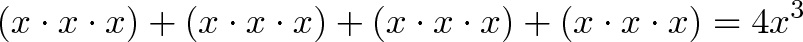$\displaystyle{(x \cdot x \cdot x) + (x \cdot x \cdot x) + (x \cdot x \cdot x) + (x \cdot x \cdot x) = 4 x^3}$

In a sentence: the derivative of x^4 is 4x^3 because x^4 has four identical "points of view" which are being combined. Booyeah!

## Take A Breather

I hope you're seeing the derivative in a new light: we have a system of parts, we wiggle our input and see how the whole thing moves. It's about combining perspectives: what does each part add to the whole?

In the follow-up article, we'll look at even more powerful rules (exponents, quotients, and friends). Happy math.

## Join 450k Monthly Readers

Enjoy the article? There's plenty more to help you build a lasting, intuitive understanding of math. Join the newsletter for bonus content and the latest updates.